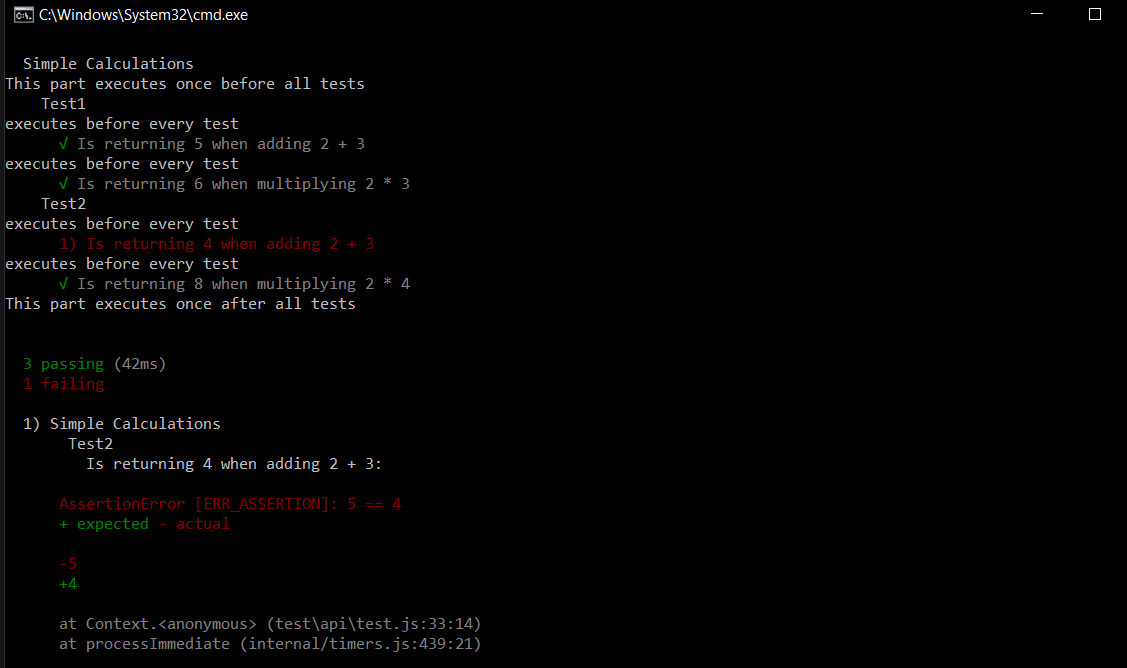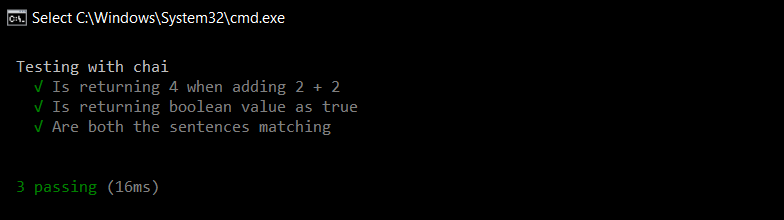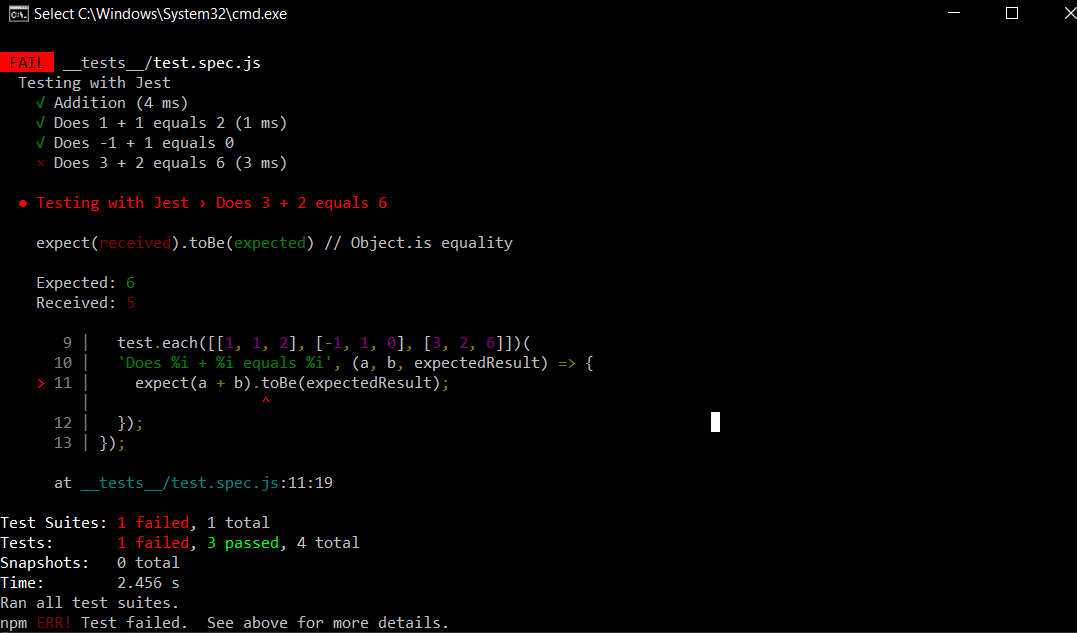# Unit Testing of Node.js Application

Node.js is a widely used javascript library based on Chrome’s V8 JavaScript engine for developing server-side applications in web development.

Unit Testing is a software testing method where individual units/components are tested in isolation. A unit can be described as the smallest testable part of code in an application. Unit testing is generally carried out by developers during the development phase of an application.

In Node.js there are many frameworks available for running unit tests. Some of them are:

• Mocha
• Jest
• Jasmine
• AVA

Unit testing for a node application using these frameworks:

1. Mocha: Mocha is an old and widely used testing framework for node applications. It supports asynchronous operations like callbacks, promises, and async/await. It is a highly extensible and customizable framework that supports different assertions and mocking libraries.

To install it, open command prompt and type the following command:

```# Installs globally
npm install mocha -g

# installs in the current directory
npm install mocha --save-dev
```

How to use Mocha?
In order to use this framework in your application:

1. Open the root folder of your project and create a new folder called test in it.
2. Inside the test folder, create a new file called test.js which will contain all the code related to testing.
3. open package.json and add the following line in the scripts block.
```"scripts": {
"test": "mocha --recursive --exit"
}```

Example:

 `// Requiring module ` `const assert = require(``'assert'``); ` ` `  `// We can group similar tests inside a describe block ` `describe(``"Simple Calculations"``, () => { ` `  ``before(() => { ` `    ``console.log( ``"This part executes once before all tests"` `); ` `  ``}); ` ` `  `  ``after(() => { ` `    ``console.log( ``"This part executes once after all tests"` `); ` `  ``}); ` `     `  `  ``// We can add nested blocks for different tests ` `  ``describe( ``"Test1"``, () => { ` `    ``beforeEach(() => { ` `      ``console.log( ``"executes before every test"` `); ` `    ``}); ` `     `  `    ``it(``"Is returning 5 when adding 2 + 3"``, () => { ` `      ``assert.equal(2 + 3, 5); ` `    ``}); ` ` `  `    ``it(``"Is returning 6 when multiplying 2 * 3"``, () => { ` `      ``assert.equal(2*3, 6); ` `    ``}); ` `  ``}); ` ` `  `  ``describe(``"Test2"``, () => { ` `    ``beforeEach(() => { ` `      ``console.log( ``"executes before every test"` `); ` `    ``}); ` `     `  `    ``it(``"Is returning 4 when adding 2 + 3"``, () => { ` `      ``assert.equal(2 + 3, 4); ` `    ``}); ` ` `  `    ``it(``"Is returning 8 when multiplying 2 * 4"``, () => { ` `      ``assert.equal(2*4, 8); ` `    ``}); ` `  ``}); ` `});`

Copy the above code and paste it in the test.js file that we have created before. To run these tests, open the command prompt in the root directory of the project and type the following command:

`npm run test`

Output:What is Chai?
Chai is an assertion library that is often used alongside Mocha. It can be used as a TTD (Test Driven Development) / BDD (Behavior Driven Development) assertion library for Node.js that can be paired up with any testing framework based on JavaScript. Similar to assert.equal() statement in the above code, we can use Chai to write tests like English sentences.

To install it, open the command prompt in the root directory of the project and type the following command:

`npm install chai`

Example:

 `const expect = require(``'chai'``).expect; ` ` `  `describe(``"Testing with chai"``, () => { ` `    ``it(``"Is returning 4 when adding 2 + 2"``, () => { ` `      ``expect(2 + 2).to.equal(4); ` `    ``}); ` ` `  `    ``it(``"Is returning boolean value as true"``, () => { ` `      ``expect(5 == 5).to.be.``true``; ` `    ``}); ` `     `  `    ``it(``"Are both the sentences matching"``, () => { ` `      ``expect(``"This is working"``).to.equal(``'This is working'``); ` `    ``}); ` ` ``}); `

Output:2. Jest: Jest is also a popular testing framework that is known for its simplicity. It is developed and maintained regularly by Facebook. One of the key features of jest is it is well documented, and it supports parallel test running i.e. each test will run in their own processes to maximize performance. It also includes several features like test watching, coverage, and snapshots.

You can install it using the following command:

`npm install --save-dev jest`

Note: By default, Jest expects to find all the test files in a folder called “__tests__” in your root folder.

Example:

 `describe(``"Testing with Jest"``, () => { ` `  ``test(``"Addition"``, () => { ` `    ``const sum = 2 + 3; ` `    ``const expectedResult = 5; ` `    ``expect(sum).toEqual(expectedResult); ` `  ``}); ` `   `  `  ``// Jest also allows a test to run multiple ` `  ``// times using different values ` `  ``test.each([[1, 1, 2], [-1, 1, 0], [3, 2, 6]])( ` `  ``'Does %i + %i equals %i'``, (a, b, expectedResult) => { ` `    ``expect(a + b).toBe(expectedResult); ` `  ``}); ` `});`

Output:3. Jasmine: Jasmine is also a powerful testing framework and has been around since 2010. It is a Behaviour Driven Development(BDD) framework for testing JavaScript code. It is known for its compatibility and flexibility with other testing frameworks like Sinon and Chai. Here test files must have a specific suffix (*spec.js).

You can install it using the following command:

`npm install jasmine-node`

Example:

 `describe(``"Test"``, ``function``() { ` `  ``it(``"Addition"``, ``function``() { ` `    ``var` `sum = 2 + 3; ` `    ``expect(sum).toEqual(5); ` `  ``}); ` `});`

4. AVA: AVA is a relatively new minimalistic framework that allows you to run your JavaScript tests concurrently. Like the Jest framework, it also supports snapshots and parallel processing which makes it relatively fast compared to other frameworks. The key features include having no implicit globals and built-in support for asynchronous functions.

You can install it using the following command:

`npm init ava`

Example:

 `import test from ``'ava'``; ` ` `  `test(``'Addition'``, t => { ` `  ``t.is(2 + 3, 5); ` `});`My Personal Notes arrow_drop_upCheck out this Author's contributed articles.

If you like GeeksforGeeks and would like to contribute, you can also write an article using contribute.geeksforgeeks.org or mail your article to contribute@geeksforgeeks.org. See your article appearing on the GeeksforGeeks main page and help other Geeks.

Please Improve this article if you find anything incorrect by clicking on the "Improve Article" button below.